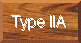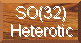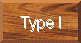# SUPERSTRINGS! Supersymmetric Strings

There are two types of particles in nature - fermions and bosons. A fundamental theory of nature must contain both of these types. When we include fermions in the worldsheet theory of the string, we automatically get a new type of symmetry called supersymmetry which relates bosons and fermions. Fermions and bosons are grouped together into supermultiplets which are related under the symmetry. This is the reason for the "super" in "superstrings".

A consistent quantum field theory of superstrings exists only in 10 spacetime dimensions! Otherwise there are quantum effects which render the theory inconsistent or 'anomalous'. In 10 spacetime dimensions the effects can precisely cancel leaving the theory anomaly free. It may seem to be a problem to have 10 spacetime dimensions instead of the 4 spacetime dimensions that we observe, but we will see that in getting from 10 to 4 we actually find some interesting physics.

In terms of weak coupling perturbation theory there appear to be only five different consistent superstring theories known as Type I SO(32), Type IIA, Type IIB, SO(32) Heterotic and E8 x E8 Heterotic.String Type Closed Closed Closed Closed Open  (& closed) 10d Supersymmetry N=2  (chiral) N=2  (non-chiral) N=1 N=1 N=1 10d Gauge groups none none E8 x E8 SO(32) SO(32) D-branes -1,1,3,5,7 0,2,4,6,8 none none 1,5,9

• Type I SO(32):

• This is a theory which contains open superstrings. It has one (N=1) supersymmetry in 10 dimensions. Open strings can carry gauge degrees of freedom at their endpoints, and cancellation of anomalies uniquely constrains the gauge group to be SO(32). It contains D-branes with 1, 5, and 9 spatial dimensions.

• Type IIA:

• This is a theory of closed superstrings which has two (N=2) supersymmetries in ten dimensions. The two gravitini (superpartners to the graviton) move in opposite directions on the closed string world sheet and have opposite chiralities under the 10 dimensional Lorentz group, it is a non-chiral theory. There is no gauge group. It contains D-branes with 0, 2, 4, 6, and 8 spatial dimensions.

• Type IIB:

• This is also a closed superstring theory with N=2 supersymmetry. However in this case the two gravitini have the same chiralities under the 10 dimensional Lorentz group, so this is a chiral theory. Again there is no gauge group, but it contains D-branes with -1, 1, 3, 5, and 7 spatial dimensions.

• SO(32) Heterotic:

• This is a closed string theory with worldsheet fields moving in one direction on the world sheet which have a supersymmetry and fields moving in the opposite direction which have no supersymmetry. The result is N=1 supersymmetry in 10 dimensions. The non-supersymmetric fields contribute massless vector bosons to the spectrum which by anomaly cancellation are required to have an SO(32) gauge symmetry.

• E8 x E8 Heterotic:

• This theory is identical to the SO(32) Heterotic string, except that the gauge group is E8 X E8 which is the only other gauge group allowed by anomaly cancellation.
We see that the Heterotic theories don't contain D-branes. They do however contain a fivebrane soliton which is not a D-brane. The IIA and IIB theories also contain this fivebrane soliton in addition to the D-branes. This fivebrane is usually called the "Neveu-Schwarz fivebrane" or "NS fivebrane".

It is worthwhile to note that the E8 x E8 Heterotic string has historically been considered to be the most promising string theory for describing the physics beyond the Standard Model.  It was discovered in 1987 by Gross, Harvey, Martinec, and Rohm and for a long time it was thought to be the only string theory relevant for describing our universe.  This is because the SU(3) x SU(2) x U(1) gauge group of the standard model can fit quite nicely within one of the E8 gauge groups.  The matter under the other E8 would not interact except through gravity, and might provide a answer to the Dark Matter problem in astrophysics.  Due to our lack of a full understanding of string theory, answers to questions such as how is supersymmetry broken and why are there only 3 generations of particles in the Standard Model have remained unanswered.  Most of these questions are related to the issue of compactification (discussed on the next page).  What we have learned is that string theory contains all the essential elements to be a successful unified theory of particle interactions, and it is virtually the only candidate which does so.  However, we don't yet know how these elements specifically come together to describe the physics that we currently observe.